# Texas Go Math Grade 3 Lesson 3.1 Answer Key Compare Fractions with the Same Denominator

Refer to our Texas Go Math Grade 3 Answer Key Pdf to score good marks in the exams. Test yourself by practicing the problems from Texas Go Math Grade 3 Lesson 3.1 Answer Key Compare Fractions with the Same Denominator.

## Texas Go Math Grade 3 Lesson 3.1 Answer Key Compare Fractions with the Same Denominator

Essential Question
How can you compare fractions with the same denominator?
When the denominators are the same, the fraction with the lesser numerator is the lesser fraction and the fraction with the greater numerator is the greater fraction. When the numerators are equal, the fractions are considered equivalent

Unlock the problem
Jeremy and Christina are each making a quilt block. Both blocks are the same size and both are made of 4 equal-size squares. $$\frac{2}{4}$$ of Jeremy’s squares are green. $$\frac{1}{4}$$ of Christina’s squares are green. Whose quilt block has more green squares?

• Circle the two fractions you need to compare.
The two fractions that you need to compare are:
$$\frac{2}{4}$$ and $$\frac{1}{4}$$

• How are the two fractions alike?
The two fractions have the same denominator but different numerators

Compare fractions of a whole.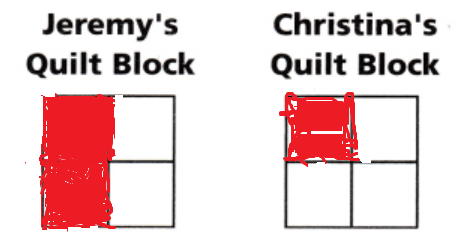• Shade $$\frac{2}{4}$$ of Jeremy’s quilt block.
• Shade $$\frac{1}{4}$$ of Christina’s quilt block.
$$\frac{2}{4}$$ > $$\frac{1}{4}$$
The greater fraction will have the larger amount of the whole shaded.
So,
Jeremy’s quilt block has more green squares.

Math Idea
You can compare two fractions when they refer to the same whole or to groups that are the same size.

Compare fractions of a group.
Jen and Maggie each have 6 buttons.

• Shade 3 of Jen’s buttons to show the number of buttons that are red. Shade 5 of Maggie’s buttons to show the number that is red.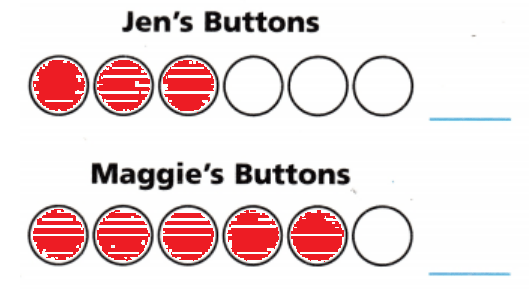• Write a fraction to show the number of red buttons in each group. Compare the fractions.
The fraction of the number of Jen’s red buttons = $$\frac{3}{6}$$
The fraction of the number of Maggie’s red buttons = $$\frac{5}{6}$$

There is the same number of buttons in each group, so you can count the number of red buttons to compare the fractions.So,
Maggie has a greater fraction of red buttons.

Use fraction strips and a number line.

At the craft store, one piece of ribbon is $$\frac{2}{8}$$ yard long. Another piece of ribbon is $$\frac{7}{8}$$ yard long. Which piece of ribbon is longer?
Compare $$\frac{2}{8}$$ and $$\frac{7}{8}$$.

• Shade the fraction strip diagrams to show the locations of $$\frac{2}{8}$$ and $$\frac{7}{8}$$.• Draw and label the points on the number line.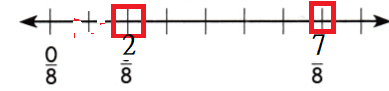• Compare the lengths.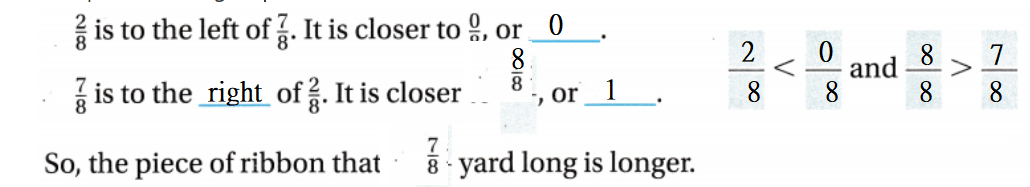Share and Show

Lesson 3.1 Compare Fractions with the Same Denominator Answer Key Question 1.
Draw points on the number line to show $$\frac{1}{6}$$ and $$\frac{5}{6}$$. Then compare the fractions.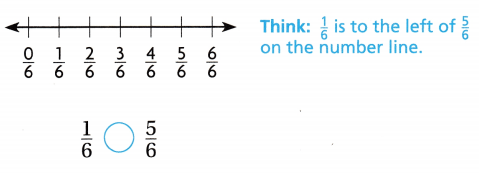The given number line is: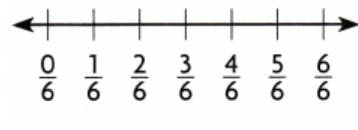Now,
When we observe the given number line,
$$\frac{1}{6}$$ is to the left of $$\frac{5}{6}$$
The denominators are the same
So,
Compare the numerators
Hence, from the above
We can conclude thatMath Talk
Mathematical Processes
Explain why fractions increase in size as you move right on the number line.
A number line is a way to see the size of numbers by placing them along a line. A number line is usually horizontal with zero in the middle. As you move to the right the numbers are positive and increase. As you go to the left, the numbers also increase and get more and more negative.

Compare. Write <, >, or =.

Question 2.
$$\frac{4}{8}$$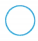$$\frac{3}{8}$$
The given fractions are:
$$\frac{4}{8}$$ and $$\frac{3}{8}$$
Now,
We know that,
If the denominators are the same, then compare the numerators
So,
4 > 3
Hence, from the above,
We can conclude that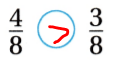Question 3.
$$\frac{1}{4}$$$$\frac{4}{4}$$
The given fractions are:
$$\frac{1}{4}$$ and $$\frac{4}{4}$$
Now,
We know that,
If the denominators are the same, then compare the numerators
So,
1 < 4
Hence, from the above,
We can conclude thatGo Math Grade 3 Lesson 3.1 Compare Fractions Answer Key Question 4.
$$\frac{1}{2}$$$$\frac{1}{2}$$
The given fractions are:
$$\frac{1}{2}$$ and $$\frac{1}{2}$$
Now,
We know that,
If the denominators are the same, then compare the numerators
So,
1 = 1
Hence, from the above,
We can conclude that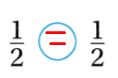Question 5.
$$\frac{3}{6}$$$$\frac{2}{6}$$
The given fractions are:
$$\frac{3}{6}$$ and $$\frac{2}{6}$$
Now,
We know that,
If the denominators are the same, then compare the numerators
So,
3 > 2
Hence, from the above,
We can conclude that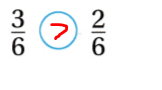Problem Solving

Question 6.
Carlos finished $$\frac{5}{8}$$ of his art project on Monday. Tyler finished $$\frac{7}{8}$$ of his art project on Monday. Who finished more of his art project on Monday?
It is given that
Carlos finished $$\frac{5}{8}$$ of his art project on Monday. Tyler finished $$\frac{7}{8}$$ of his art project on Monday
Now,
The given fractions are:
$$\frac{5}{8}$$ and $$\frac{7}{8}$$
Now,
We know that,
If the denominators are the same, then compare the numerators
So,
5 < 7
So,
$$\frac{5}{8}$$ < $$\frac{7}{8}$$
Hence, from the above,
We can conclude that
Tyler finished more of his art project on Monday

Question 7.
Mallory picked 4 roses for her mother. One-fourth of the roses are pink and $$\frac{3}{4}$$ of the roses are red. Are there more pink roses or red roses?
It is given that
Mallory picked 4 roses for her mother. One-fourth of the roses are pink and $$\frac{3}{4}$$ of the roses are red
So,
The fraction of the roses that are pink = $$\frac{1}{4}$$
Now,
The given fractions are:
$$\frac{1}{4}$$ and $$\frac{3}{4}$$
Now,
We know that,
If the denominators are the same, then compare the numerators
So,
1 < 3
So,
$$\frac{1}{4}$$ < $$\frac{3}{4}$$
Hence, from the above,
We can conclude that
There are more red roses

Use Tools Multi-Step Lauren ran $$\frac{7}{8}$$ of a mile in a race. Jacob ran $$\frac{5}{8}$$ of a mile. Draw points on the number line to show $$\frac{7}{8}$$ and $$\frac{5}{8}$$. Then compare the fractions.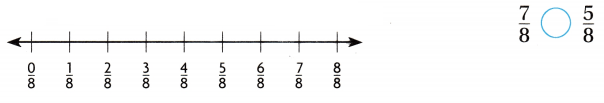It is given that
Lauren ran $$\frac{7}{8}$$ of a mile in a race. Jacob ran $$\frac{5}{8}$$ of a mile
Now,
The given number line is:Now,
The representation of the number of miles on the given number line is: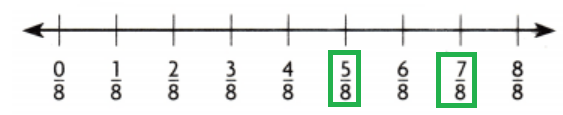Now,
The given fractions are:
$$\frac{5}{8}$$ and $$\frac{7}{8}$$
Now,
We know that,
If the denominators are the same, then compare the numerators
So,
5 < 7
So,
$$\frac{5}{8}$$ < $$\frac{7}{8}$$
Hence, from the above,
We can conclude that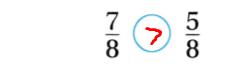Question 9.
H.O.T. What’s the Error? Gary and Vanessa are comparing fractions. Vanessa models $$\frac{2}{4}$$ and Gary models $$\frac{3}{4}$$. Vanessa writes $$\frac{3}{4}$$ < $$\frac{2}{4}$$. Look at Gary’s model and Vanessa’s model and find her error.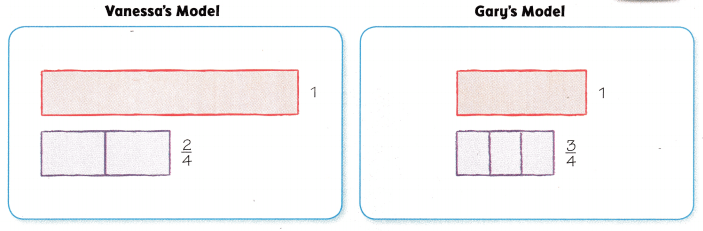Multi-Step Describe Vanessa’s error and explain how to correct it.
It is given that
Gary and Vanessa are comparing fractions. Vanessa models $$\frac{2}{4}$$ and Gary models $$\frac{3}{4}$$. Vanessa writes $$\frac{3}{4}$$ < $$\frac{2}{4}$$
Now,
The given models are:Now,
From the above models,
We can observe that
The total number of parts is the same
The number of shaded parts is different
Now,
The given fractions are:
$$\frac{2}{4}$$ and $$\frac{3}{4}$$
Now,
We know that,
If the denominators are the same, then compare the numerators
So,
2 < 3
So,
$$\frac{2}{4}$$ < $$\frac{3}{4}$$
Hence, from the above,
We can conclude that
Vanessa’s error is: The incorrect counting of the number of shaded boxes
The correct comparison is:
$$\frac{2}{4}$$ < $$\frac{3}{4}$$

Use fraction strips to compare. Fill in the bubble for the correct answer choice.

Question 10.
At a car show, $$\frac{4}{8}$$ of the cars are red. A smaller fraction of the cars is black. Which could be the fraction of the cars that are black?
(A) $$\frac{4}{8}$$
(B) $$\frac{2}{8}$$
(C) $$\frac{8}{8}$$
(D) $$\frac{6}{8}$$
It is given that
At a car show, $$\frac{4}{8}$$ of the cars are red. A smaller fraction of the cars is black.
Now,
Let the total number of cars be: 1
So,
(The fraction of cars that are red) + (The fraction of cars that are black) = 1
So,
The number of cars that are black = 1 – $$\frac{4}{8}$$
= $$\frac{8 – 4}{8}$$
= $$\frac{4}{8}$$
Hence, from the above,
We can conclude that
The fraction of the cars that are black are:Question 11.
Which fraction makes the statement true?(A) $$\frac{3}{6}$$
(B) $$\frac{2}{6}$$
(C) $$\frac{5}{6}$$
(D) $$\frac{1}{6}$$
The given fraction is:
$$\frac{2}{6}$$
Now,
We know that,
If the denominators are the same, compare the numerators
Hence, from the above,
We can conclude that
The fraction that makes the given statement true is: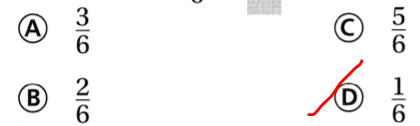Apply Multi-Step There are two same-size scarves. Jo’s scarf is divided into 4 equal sections. Three of the sections are blue. A greater fraction of Morgan’s scarf is blue. Which could be the fraction of Morgan’s scarf that is blue?
(A) $$\frac{4}{4}$$
(B) $$\frac{2}{4}$$
(C) $$\frac{1}{4}$$
(D) $$\frac{3}{4}$$
It is given that
There are two same-size scarves. Jo’s scarf is divided into 4 equal sections. Three of the sections are blue. A greater fraction of Morgan’s scarf is blue
Now,
According to the given information,
The fraction of Morgan’s scarf that is blue = (The number of sections that are shaded blue) ÷ (The total number of sections)
= $$\frac{3}{4}$$
Hence, from the above,
We can conclude that
The fraction of Morgan’s scarf that is blue is:Texas Test Prep

Question 13.
Todd and Lisa are comparing fraction strips. Which statement is NOT correct?
(A) $$\frac{1}{4}$$ < $$\frac{4}{4}$$
(B) $$\frac{5}{6}$$ < $$\frac{4}{6}$$ (C) $$\frac{2}{3}$$ > $$\frac{1}{3}$$
(D) $$\frac{5}{8}$$ > $$\frac{4}{8}$$
It is given that
Todd and Lisa are comparing fraction strips
Hence, from the above,
We can conclude that
The statement that is not correct is: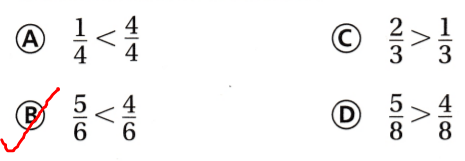### Texas Go Math Grade 3 Lesson 3.1 Homework and Practice Answer Key

Compare. Write <, >, or =.

Question 1.
$$\frac{5}{8}$$$$\frac{4}{8}$$
The given fractions are:
$$\frac{5}{8}$$ and $$\frac{4}{8}$$
Now,
We know that,
If the denominators are the same, then compare the numerators
So,
5 > 4
Hence, from the above,
We can conclude that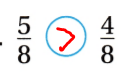Question 2.
$$\frac{1}{4}$$$$\frac{4}{4}$$
The given fractions are:
$$\frac{1}{4}$$ and $$\frac{4}{4}$$
Now,
We know that,
If the denominators are the same, then compare the numerators
So,
1 < 4
Hence, from the above,
We can conclude that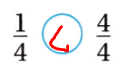Question 3.
$$\frac{1}{6}$$$$\frac{2}{6}$$
The given fractions are:
$$\frac{1}{6}$$ and $$\frac{2}{6}$$
Now,
We know that,
If the denominators are the same, then compare the numerators
So,
1 < 2
Hence, from the above,
We can conclude that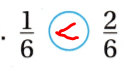Question 4.
$$\frac{2}{3}$$$$\frac{1}{3}$$
The given fractions are:
$$\frac{2}{3}$$ and $$\frac{1}{3}$$
Now,
We know that,
If the denominators are the same, then compare the numerators
So,
2 > 1
Hence, from the above,
We can conclude that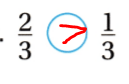Question 5.
$$\frac{1}{2}$$$$\frac{0}{2}$$
The given fractions are:
$$\frac{1}{2}$$ and $$\frac{0}{2}$$
Now,
We know that,
If the denominators are the same, then compare the numerators
So,
1 > 0
Hence, from the above,
We can conclude that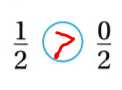Question 6.
$$\frac{7}{8}$$$$\frac{6}{8}$$
The given fractions are:
$$\frac{7}{8}$$ and $$\frac{6}{8}$$
Now,
We know that,
If the denominators are the same, then compare the numerators
So,
7 > 6
Hence, from the above,
We can conclude that$$\frac{5}{6}$$$$\frac{5}{6}$$
The given fractions are:
$$\frac{5}{6}$$ and $$\frac{5}{6}$$
Now,
We know that,
If the denominators are the same, then compare the numerators
So,
5 = 5
Hence, from the above,
We can conclude thatQuestion 8.
$$\frac{1}{3}$$$$\frac{3}{3}$$
The given fractions are:
$$\frac{1}{3}$$ and $$\frac{3}{3}$$
Now,
We know that,
If the denominators are the same, then compare the numerators
So,
1 < 3
Hence, from the above,
We can conclude thatProblem Solving

Question 9.
Greg finished $$\frac{3}{8}$$ of his chores on Saturday and $$\frac{5}{8}$$ of his chores on Sunday. On which day did he finish fewer chores?
It is given that
Greg finished $$\frac{3}{8}$$ of his chores on Saturday and $$\frac{5}{8}$$ of his chores on Sunday
Now,
The given fractions are:
$$\frac{3}{8}$$ and $$\frac{5}{8}$$
Now,
We know that,
If the denominators are the same, then compare the numerators
So,
3 < 5
So,
$$\frac{3}{8}$$ < $$\frac{5}{8}$$
Hence, from the above,
We can conclude that
Greg finished the least chores on Saturday

Question 10.
Tia completed $$\frac{3}{6}$$ of her math homework problems before dinner. After dinner, she finished $$\frac{3}{6}$$ of the problems. Did she do more problems before or after dinner?
It is given that
Tia completed $$\frac{3}{6}$$ of her math homework problems before dinner. After dinner, she finished $$\frac{3}{6}$$ of the problems
Now,
The given fractions are:
$$\frac{3}{6}$$ and $$\frac{3}{6}$$
Now,
We know that,
If the denominators are the same, then compare the numerators
So,
3 = 3
So,
$$\frac{3}{6}$$ = $$\frac{3}{6}$$
Hence, from the above,
We can conclude that
Tia did the same problems before and after dinner

Texas test Prep

Lesson Check

Question 11.
Which fraction makes the statement true?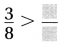(A) $$\frac{7}{8}$$
(B) $$\frac{5}{8}$$
(C) $$\frac{2}{8}$$
(D) $$\frac{4}{8}$$
The given fraction is:
$$\frac{3}{8}$$
Now,
We know that,
If the denominators are the same, then compare the numerators
Hence, from the above,
We can conclude that
The fraction that makes the given statement true is: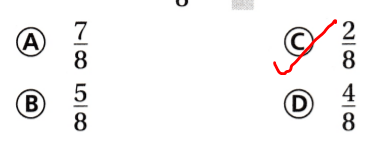Question 12.
Which fraction makes the statement true?(A) $$\frac{1}{6}$$
(B) $$\frac{3}{6}$$
(C) $$\frac{5}{6}$$
(D) $$\frac{2}{6}$$
The given fraction is:
$$\frac{3}{6}$$
Now,
We know that,
If the denominators are the same, then compare the numerators
Hence, from the above,
We can conclude that
The fraction that makes the given statement true is:Question 13.
In a fish tank, $$\frac{5}{8}$$ of the fish are yellow. A smaller fraction of the fish is blue. Which could be the fraction of the fish that are blue?
(A) $$\frac{3}{8}$$
(B) $$\frac{6}{8}$$
(C) $$\frac{8}{8}$$
(D) $$\frac{5}{8}$$
It is given that
In a fish tank, $$\frac{5}{8}$$ of the fish are yellow. A smaller fraction of the fish is blue
Now,
Let the total number of fish in a fish tank be: 1
So,
(The fraction of the fish that are yellow) + (The fraction of the fish that are blue) = 1
So,
The fraction of the fish that are blue = 1 – $$\frac{5}{8}$$
= $$\frac{8 – 5}{8}$$
= $$\frac{3}{8}$$
Hence, from the above,
We can conclude that
The fraction of the fish that is blue is:Question 14.
Wes collects sports cards. In his collection, $$\frac{3}{6}$$ of the cards are baseball cards. A smaller fraction of the cards is football cards. Which could be the fraction of the cards that are football cards?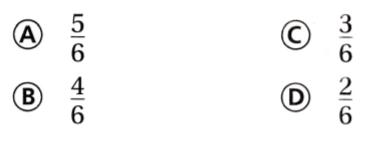It is given that
Wes collects sports cards. In his collection, $$\frac{3}{6}$$ of the cards are baseball cards. A smaller fraction of the cards is football cards
Now,
Let the total number of sports cards be: 1
So,
(The fraction of the cards that is baseball cards) + (The fraction of the cards that is football cards) = 1
So,
The fraction of the cards that is football cards = 1 – $$\frac{3}{6}$$
= $$\frac{6 – 3}{6}$$
= $$\frac{3}{6}$$
Hence, from the above,
We can conclude that
The fraction of the cards that is football cards is: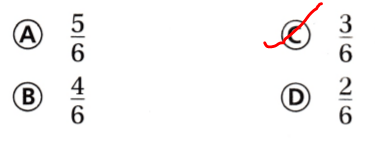Multi-Step Gerri and Jose are modeling fractions. Gerri shades two of four equal parts. Jose models a fraction that is less than Gerfi’s fraction. Which could be the fraction that Jose models?
(A) $$\frac{3}{4}$$
(B) $$\frac{1}{4}$$
(C) $$\frac{2}{4}$$
(D) $$\frac{4}{4}$$
It is given that
Gerri and Jose are modeling fractions. Gerri shades two of four equal parts. Jose models a fraction that is less than Gerfi’s fraction
Now,
According to the given information,
The fraction that is modeled by Gerri = $$\frac{2}{4}$$
Now,
We know that,
If the denominators are the same, then the numerators must be compared
Hence, from the above,
We can conclude that
The fraction that Jose modeled is:Question 16.
Multi-Step Mr. Ames baked a slice of banana bread and blueberry bread. The family ate $$\frac{1}{4}$$ of the banana bread and $$\frac{3}{4}$$ of the blueberry bread. Which bread had more leftover? What fraction of that bread was left over?
(A) blueberry bread, $$\frac{1}{4}$$
(B) blueberry bread, $$\frac{2}{4}$$
(C) banana bread, $$\frac{3}{4}$$
(D) banana bread, $$\frac{2}{4}$$
It is given that
Mr. Ames baked a slice of banana bread and blueberry bread. The family ate $$\frac{1}{4}$$ of the banana bread and $$\frac{3}{4}$$ of the blueberry bread
Now,
The given fractions are:
$$\frac{1}{4}$$ and $$\frac{3}{4}$$
Now,
We know that,
if the denominators are the same, then the numerators must be compared
So,
1 < 3
So,
$$\frac{1}{4}$$ < $$\frac{3}{4}$$
So,
The fraction of the leftover of Banana bread = 1 – $$\frac{1}{4}$$
= $$\frac{4 – 1}{4}$$
= $$\frac{3}{4}$$